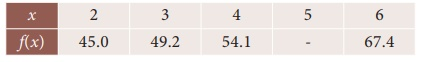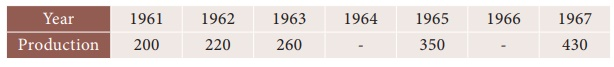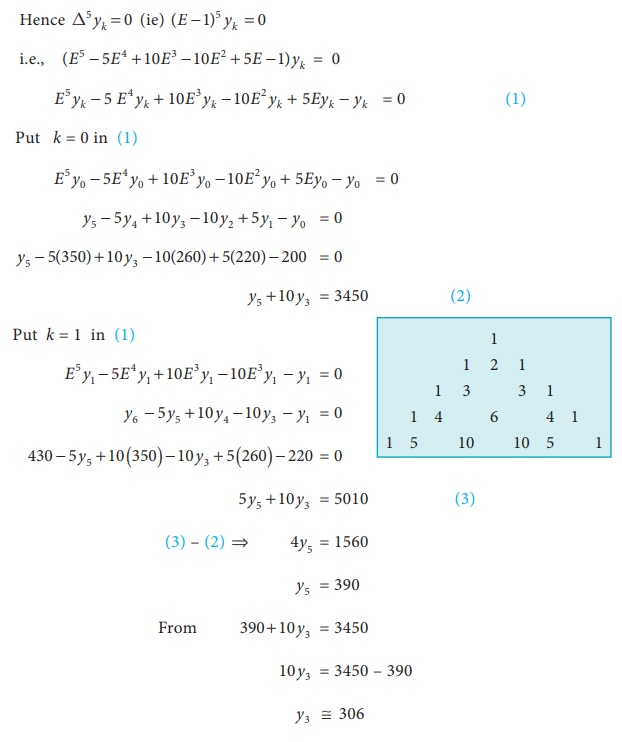Home | | Business Maths 12th Std | Finite Differences operators: Finding the missing terms

# Finite Differences operators: Finding the missing terms

Using the difference operators and shifting operator we can able to find the missing terms. Forward Difference Operator(âˆ†), Backward Difference operator(âˆ‡), Shifting operator (E)

Finding the missing terms

Using the difference operators and shifting operator we can able to find the missing terms.

Example 5.10

From the following table find the missing valueSolution:

Since only four values of f(x) are given, the polynomial which fits the data is of degree three. Hence fourth differences are zeros.Example 5.11

Estimate the production for 1964 and 1966 from the following dataSolution:

Since five values are given, the polynomial which fits the data is of degree four.Tags : Example Solved Problems with Answer, Solution, Formula , 12th Business Maths and Statistics : Chapter 5 : Numerical Methods
Study Material, Lecturing Notes, Assignment, Reference, Wiki description explanation, brief detail
12th Business Maths and Statistics : Chapter 5 : Numerical Methods : Finite Differences operators: Finding the missing terms | Example Solved Problems with Answer, Solution, Formula